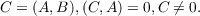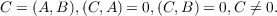#### Vol. 20, No. 2, 1967

 Download this articleFor screen For printingRecent Issues Vol. 325: 1  2 Vol. 324: 1  2 Vol. 323: 1  2 Vol. 322: 1  2 Vol. 321: 1  2 Vol. 320: 1  2 Vol. 319: 1  2 Vol. 318: 1  2Online Archive Volume: Issue:The Journal Subscriptions Editorial Board Officers Contacts Submission Guidelines Submission Form Policies for Authors ISSN: 1945-5844 (e-only) ISSN: 0030-8730 (print) Special Issues Author Index To Appear Other MSP Journals
On a class of matrix equations

### Robert Charles Thompson

Vol. 20 (1967), No. 2, 289–316
##### Abstract

Let K be a field of characteristic p,p zero or prime, and let L be the algebraic closure of K. Let Mn(K) denote the matrix algebra of n-square matrices with elements in K. The commutator of A,B Mn(K) is defined by (A,B) = AB BA. It is the object of this paper to examine the following two questions.

I. Given exactly one of the three matrices A,B,C Mn(K), to determine necessary and sufficient conditions in order that the other two matrices will exist in Mn(K) such that(1)

II. Given exactly one of the three matrices A,B,C Mn(K), to determine necessary and sufficient conditions in order that the other two matrices will exist in Mn(K) such that(2)

We shall obtain complete solutions to all these problems, except that, in Question I when C is the given matrix and 0 < p n, we obtain only a partial solution. As a consequence of our results, we are able to find conditions that are sufficient, and sometimes necessary and sufficient, in order that solutions exist in Mn(K) for certain complicated families of commutator equations related to (1) or (2).

Primary: 15.35
Secondary: 16.48
##### Milestones